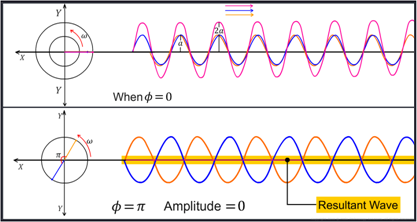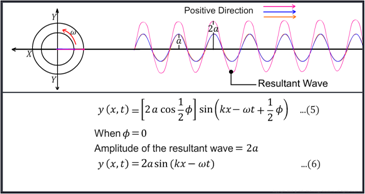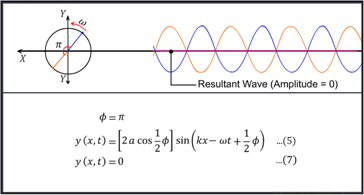Notes On Principle of Superposition of Waves - CBSE Class 11 Physics
Let us consider two pulses travelling simultaneously along the same stretched string in opposite directions. The net displacement of any element of the string as the two pulses cross each other is the algebraic sum of the displacements due to each pulse. After crossing each other, the pulses move forward unchanged.   The addition of the wave forms or pulses to find the net or resultant wave form is known as the Principle of Superposition.     Y 1 (X, t) represents the displacement of any element of the string at time t due to the first wave. Y 2 (X, t) represents the displacement of any element of the string at time t due to the second wave.   Let us now consider two waves travelling simultaneously along the same stretched string in opposite directions. When the two waves overlap we have Y (X, t) representing the displacement of the string in the overlap region at time t.   Mathematically, we can write this as:   Y (X, t) = Y 1 (X, t) + Y 2 (X, t).   This expression implies that overlapping waves algebraically add up to produce the resultant wave.   Let us consider an example to observe the effect of the superposition of waves.   When the waves are propagating in the same direction along a stretched string: Both the waves have the same angular frequency w and angular wave number K as they are propagating on the same string.   2. They have the same amplitude A.   3. Their phases at a given x and t differ by a constant anglef.By changing the angle (f), we can observe different net wave forms. That is: When f is equal to zero, we find that the amplitude of the net wave form is doubled.   2.  When f is equal to π, the two waves are completely out of phase and therefore the amplitude of the net wave form reduces to zero and we get a straight line.   3.   When the value of f is between zero and π the amplitude of the net wave form keeps changing from 2 A to zero where ‘A’ is the amplitude of the original wave form. The net wave form shifts by f/2.These observations are expressed as the followingSummary

Let us consider two pulses travelling simultaneously along the same stretched string in opposite directions. The net displacement of any element of the string as the two pulses cross each other is the algebraic sum of the displacements due to each pulse. After crossing each other, the pulses move forward unchanged.   The addition of the wave forms or pulses to find the net or resultant wave form is known as the Principle of Superposition.     Y 1 (X, t) represents the displacement of any element of the string at time t due to the first wave. Y 2 (X, t) represents the displacement of any element of the string at time t due to the second wave.   Let us now consider two waves travelling simultaneously along the same stretched string in opposite directions. When the two waves overlap we have Y (X, t) representing the displacement of the string in the overlap region at time t.   Mathematically, we can write this as:   Y (X, t) = Y 1 (X, t) + Y 2 (X, t).   This expression implies that overlapping waves algebraically add up to produce the resultant wave.   Let us consider an example to observe the effect of the superposition of waves.   When the waves are propagating in the same direction along a stretched string: Both the waves have the same angular frequency w and angular wave number K as they are propagating on the same string.   2. They have the same amplitude A.   3. Their phases at a given x and t differ by a constant anglef.By changing the angle (f), we can observe different net wave forms. That is: When f is equal to zero, we find that the amplitude of the net wave form is doubled.   2.  When f is equal to π, the two waves are completely out of phase and therefore the amplitude of the net wave form reduces to zero and we get a straight line.   3.   When the value of f is between zero and π the amplitude of the net wave form keeps changing from 2 A to zero where ‘A’ is the amplitude of the original wave form. The net wave form shifts by f/2.These observations are expressed as the followingNext